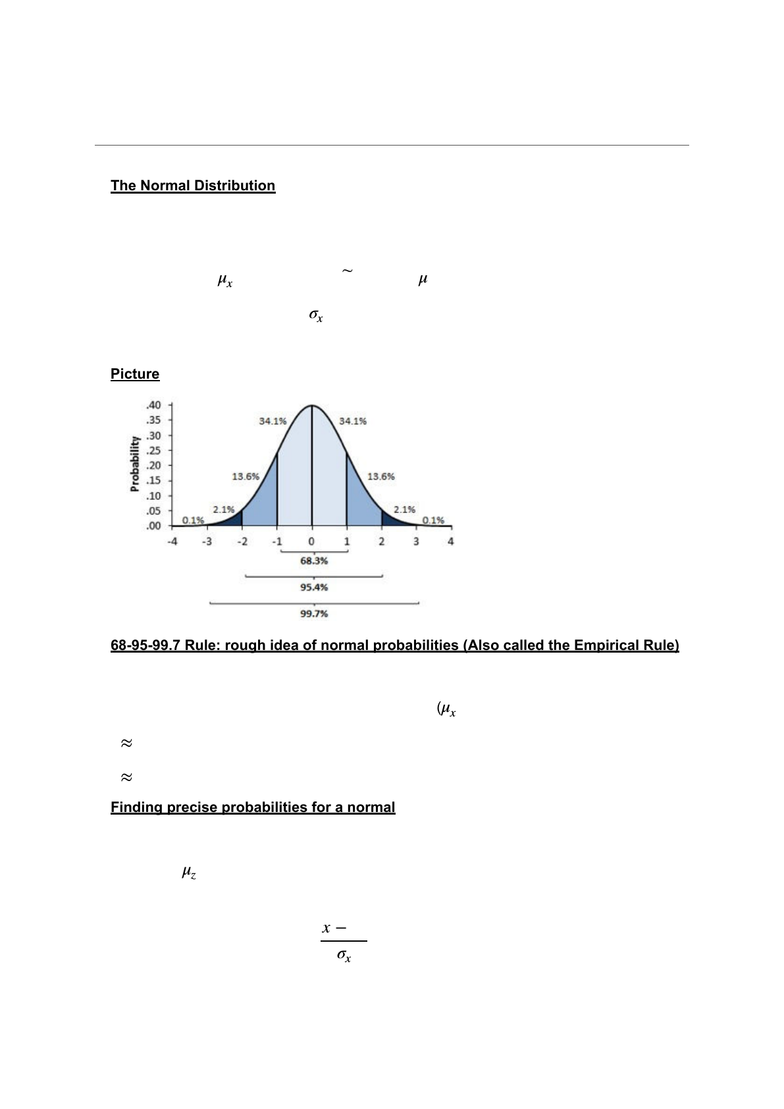# STAT 1430 Lecture Notes - Lecture 18: Standard Deviation, Random Variable, Percentile

85 views3 pagesMarch 7, 2019
STAT1430.01—Lecture 18—Normal Distribution
-The Normal Distribution
Type of variable: Continuous
Shape: Symmetric (bell-shaped)
Center: mean ! (Symmetric, so ! Median = ! Mean)
-Example: Scores, times, weights, heights
-Picture
!
-68-95-99.7 Rule: rough idea of normal probabilities (Also called the Empirical Rule)
If the values have a normal distribution:
1) =68% lie within 1 standard deviation of the mean (!)
2) !95% within 2 standard deviation of the mean
3) !99.7% within 3 standard deviation of the mean
-Finding precise probabilities for a normal
Standard normal (Z) distribution
-Mean !=0
-standard deviation is 1
-Values called z-scores !
μx
˜μ
μ
σx
μx
z=xμx
σx
1
Unlock document

This preview shows page 1 of the document.
Unlock all 3 pages and 3 million more documents.

## Get access

\$10 USD/m
Billed \$120 USD annuallyHomework Help
Study Guides
Textbook Solutions
Class Notes
Textbook Notes
Booster Class